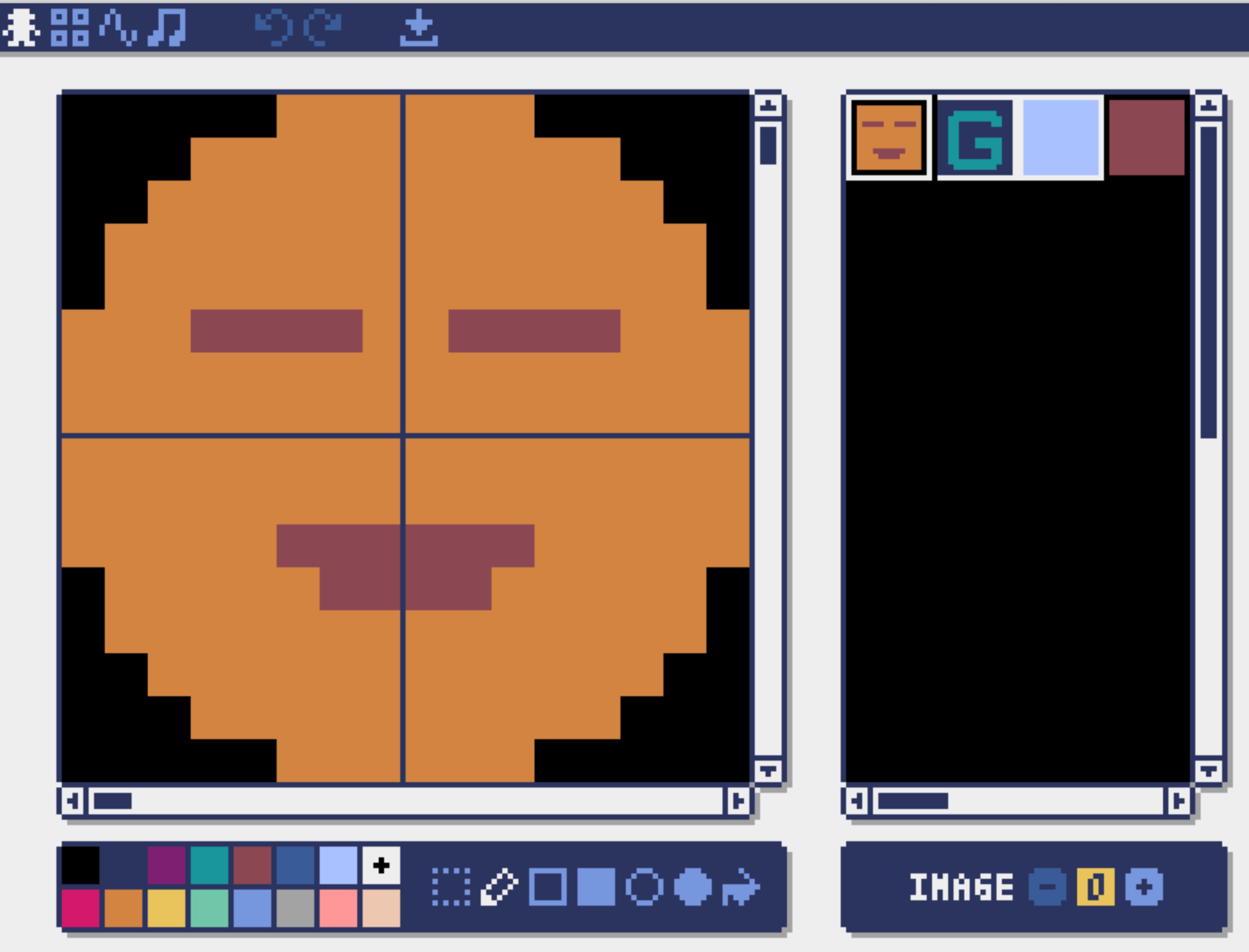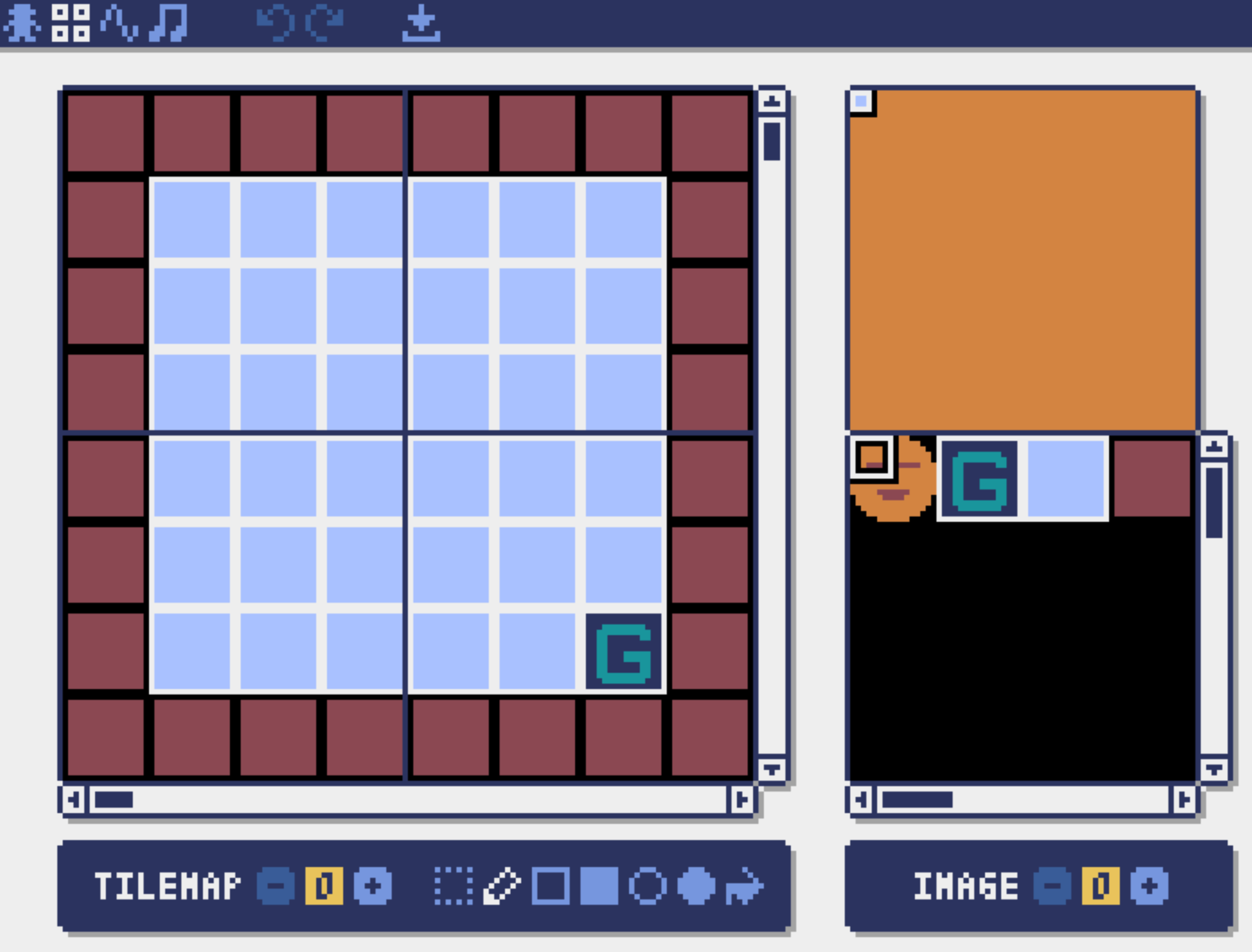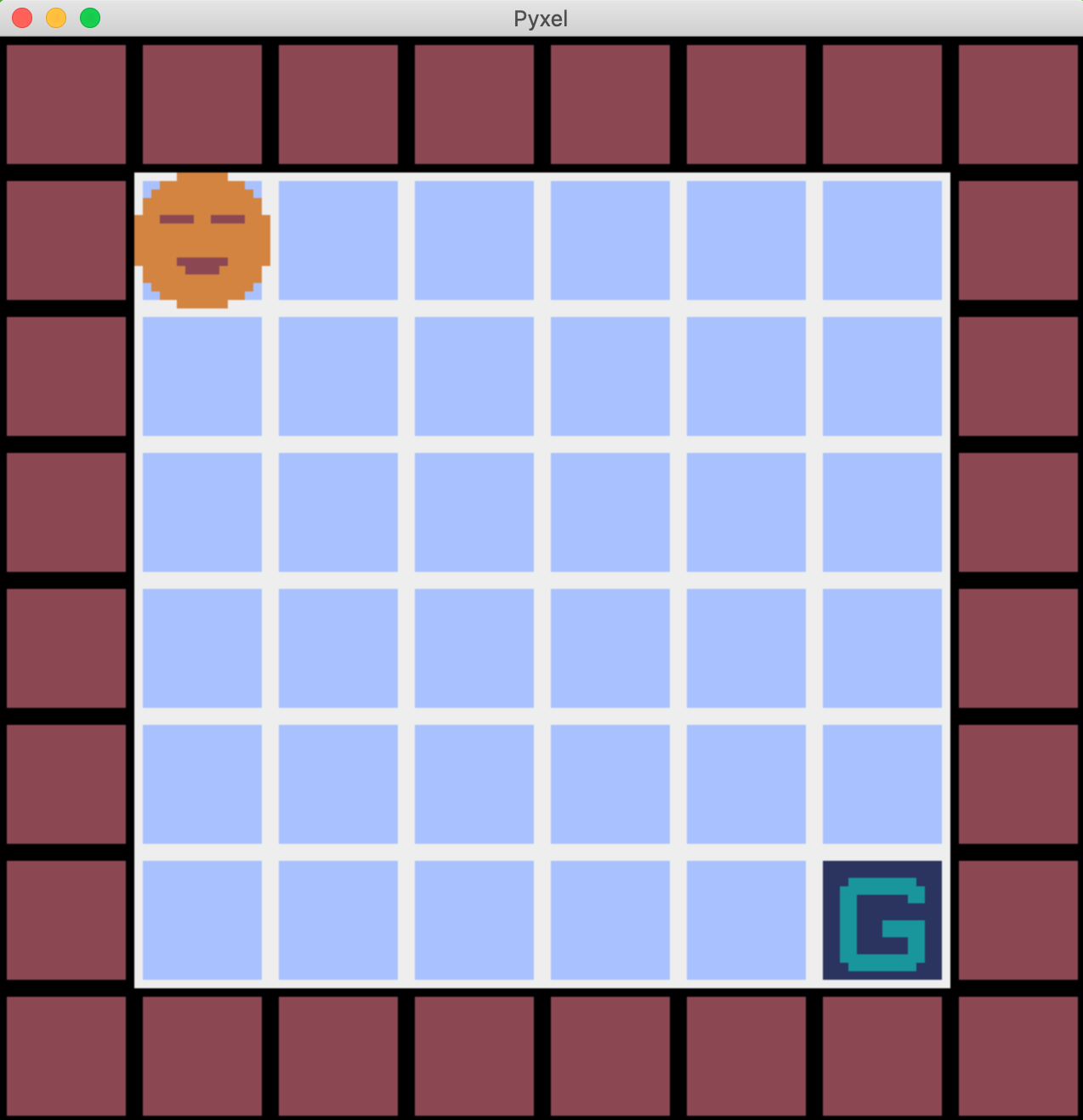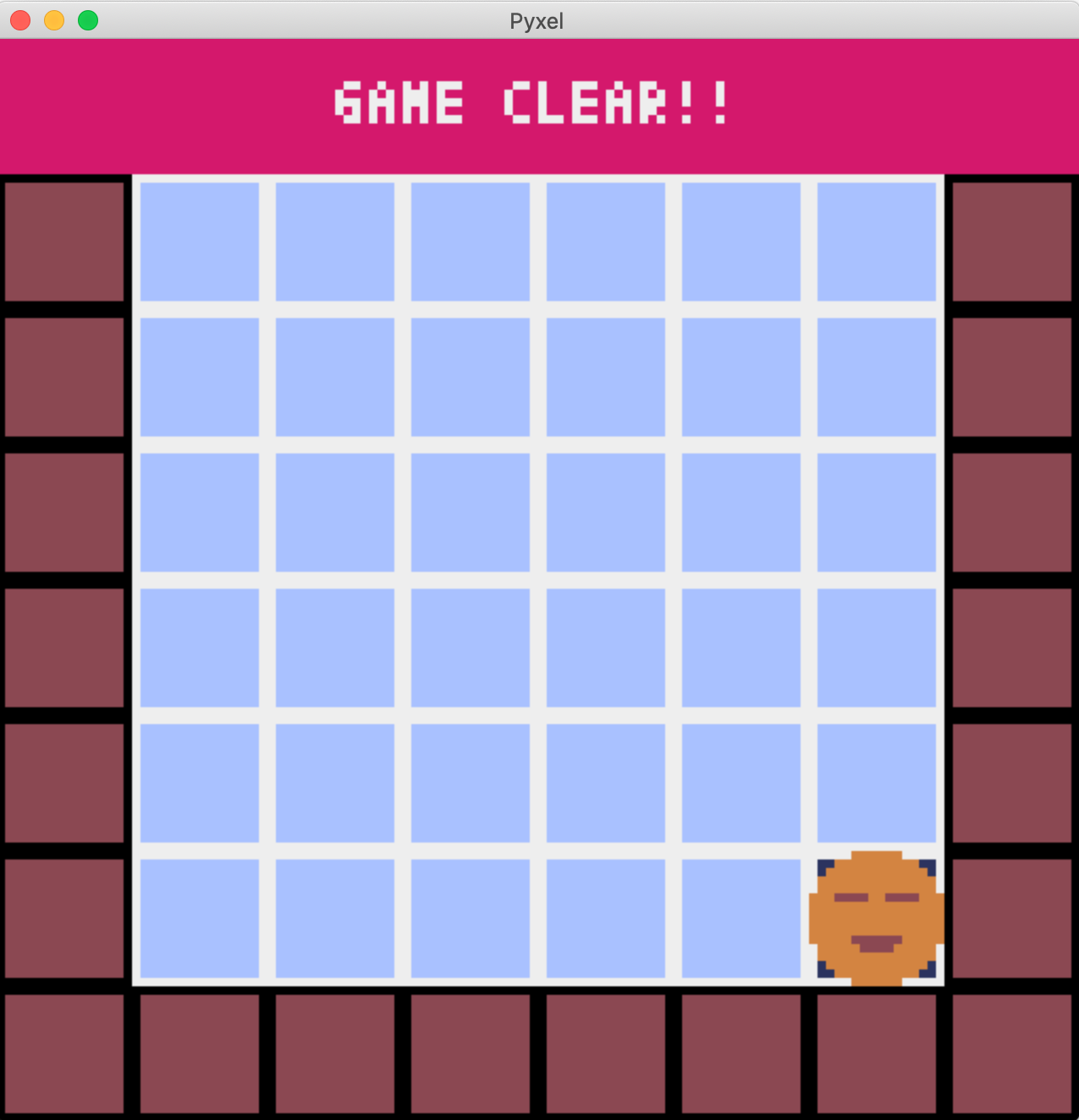# Introduction

Now that I can draw pixel art using Pyxel's editor, I'll try to make an easy game using that picture.

# Preparation

Use the image editor and tile map editor to prepare the following picture.We will create a game that moves the leftmost frowning character to the goal point marked "G". The first code is below.

``````import pyxel

#Create a character class
class Player:
def __init__(self, x, y):
#A variable that indicates the coordinates of the display position
self.x = x
self.y = y

class App:
def __init__(self):
pyxel.init(128, 128)

#Create an instance of Player class and pass the coordinates of the initial position as an argument
self.player = Player(16, 16)

def run(self):
pyxel.run(self.update, self.draw)

def update(self):
pass

def draw(self):
pyxel.cls(0)

pyxel.bltm(0, 0, 0, 0, 0, 16, 16, 0)

#player variable x,Draw using y
pyxel.blt(self.player.x, self.player.y, 0, 0, 0, 16, 16, 0)

App().run()
``````

Create a `Player` class that means a character, and define a variable that indicates the display position as a variable. The variable is used to specify the position and draw.Since the display position is set to (16, 16), it is displayed in the place where each square is moved from the left end to the lower right.

# Move the character

Let's move the character by changing the variables `x, y` of` player` according to the key input. The contents related to the change of the situation are written in the `update` function.

``````    def update(self):
#Change the display position according to the key input
if pyxel.btnp(pyxel.KEY_LEFT):
self.player.x -= 16
elif pyxel.btnp(pyxel.KEY_RIGHT):
self.player.x += 16
elif pyxel.btnp(pyxel.KEY_UP):
self.player.y -= 16
elif pyxel.btnp(pyxel.KEY_DOWN):
self.player.y += 16
``````

By increasing / decreasing `x, y` by 16 which corresponds to one square according to the key input of up / down / left / right, it is now possible to move along the square. But there are two problems here.

1. The movement ends in an instant. I want to move it a little more slowly.
2. The brown square on the outside is supposed to be a wall, but the character can slip through the wall and move off the screen.

# Move slowly

First, for 1, we will deal with it by creating a new variable that indicates the amount of movement. When the key is pressed, the movement amount is set to 16, and the movement amount is reduced each time one dot is moved. When the amount of movement reaches 0, the movement is complete. First, make the `Player` class as follows.

``````class Player:
def __init__(self, x, y):
self.x = x
self.y = y

# x,The amount of change in y and the total amount of movement
self.dx = 0
self.dy = 0
self.move_count = 0

# x,The amount of change in y x,Function to increase or decrease y
def move(self):
self.x += self.dx
self.y += self.dy
``````

Next, add the following function to the `App` class.

``````    #Function to update information about movement
def update_move(self):
#If the amount of movement is 0, it is determined whether there is a key input.
if self.player.move_count == 0:
if pyxel.btnp(pyxel.KEY_LEFT):
self.player.dx = -1
elif pyxel.btnp(pyxel.KEY_RIGHT):
self.player.dx = 1
elif pyxel.btnp(pyxel.KEY_UP):
self.player.dy = -1
elif pyxel.btnp(pyxel.KEY_DOWN):
self.player.dy = 1

#There is a key input x,If the amount of change in y is set, the amount of movement 16 is set.
if self.player.dx != 0 or self.player.dy != 0:
self.player.move_count = 16

#If the amount of movement is not 0, the state of moving
else:
#Move the player and reduce the amount of movement by 1.
self.player.move()
self.player.move_count -= 1

#When the amount of movement reaches 0, the movement ends, x,Reset the amount of change in y
if self.player.move_count == 0:
self.player.dx = 0
self.player.dy = 0
``````

Finally, write the function you just added inside the `update` function and you're done.

``````    def update(self):
self.update_move()
``````

Now you can move slowly in response to keystrokes. The point is that the situation of the character is judged as follows, and each variable is changed accordingly.

Is the character moving? → Not moved → Is there key input? → Yes / No → Moving → Has the move been completed? → Finished / Not finished

# Don't slip through the wall

Next, for 2, we will respond by determining the coordinates of the character's destination. Make the `update_move` function created earlier as follows.

``````    def update_move(self):
if self.player.move_count == 0:
if pyxel.btnp(pyxel.KEY_LEFT):
self.player.dx = -1
elif pyxel.btnp(pyxel.KEY_RIGHT):
self.player.dx = 1
elif pyxel.btnp(pyxel.KEY_UP):
self.player.dy = -1
elif pyxel.btnp(pyxel.KEY_DOWN):
self.player.dy = 1
if self.player.dx != 0 or self.player.dy != 0:
self.player.move_count = 16

#If the destination is a wall, set each variable to 0 and cancel the move
if pyxel.tilemap(0).get(
self.player.x / 8 + self.player.dx * 2,
self.player.y / 8 + self.player.dy * 2) == 6:
self.player.dx = 0
self.player.dy = 0
self.player.move_count = 0

else:
self.player.move()
self.player.move_count -= 1
if self.player.move_count == 0:
self.player.dx = 0
self.player.dy = 0
``````

I'm using the `get` function of the` tilemap` class, which is quite confusing. First, since we are using the first tilemap, the index for `tilemap` will be 0. Next, in the argument of `get`, specify the coordinates` x, y` on the tile map. It should be noted that the coordinates of the image are in dot units, and the coordinates of the tile map are 8 x 8 dots, which is 1 unit (1 tile). Here's how to convert the coordinates in dots to the coordinates in tiles.

The destination coordinates (dot unit) as an image of `player` are as follows.

``````(self.player.x + self.player.dx * 16,
self.player.y + self.player.dy * 16)
``````

It means that the current coordinates + the amount of change for each of x and y x 16 dots. Since the coordinates of the tile map are 8 dots and 1 square, x and y of the above coordinates are each divided by 8.

``````(self.player.x / 8 + self.player.dx * 2,
self.player.y / 8 + self.player.dy * 2)
``````

Now you know the coordinates for each tile.

When you enter the coordinates in this way, the "value" of the tile map that exists at those coordinates is returned. What kind of value is returned is the index of the tile in the lower right frame of the tile map editor. Since there is one tile with 8 x 8 dots, the upper left is 0, and every time the tile shifts by 8 dots to the right, it increases to 1, 2, and so on. There are 32 tiles per row, so the index is up to 31. The second line is indexed as 32,33, ..., the third line is indexed as 64,65, ... The tile that is the wall this time is the 4th tile, but if you consider 8 dots as one unit, the index will be 6. Therefore, when the return value of `get` is 6, each variable is reset to cancel the move. With the above, the movement of the character has been implemented.

# Send a message when you reach the goal

To get a message when you reach the goal, use the `draw` function as follows:

``````    def draw(self):
pyxel.cls(0)

pyxel.bltm(0, 0, 0, 0, 0, 16, 16, 0)

pyxel.blt(self.player.x, self.player.y, 0, 0, 0, 16, 16, 0)

#Send a message when you reach the goal
if pyxel.tilemap(0).get(self.player.x / 8,self.player.y / 8) == 2:
pyxel.rect(0, 0, 128, 16, 8)
pyxel.text(40, 5, "GAME CLEAR!!", 7)
``````

As before, we use the `get` function to determine if we have reached the goal. The index of the tile at the goal point is 2. You can display characters with `text`. The arguments are display position coordinates x, y, string, and color.# in conclusion

The full code.

``````import pyxel

class Player:
def __init__(self, x, y):
self.x = x
self.y = y

self.dx = 0
self.dy = 0
self.move_count = 0

def move(self):
self.x += self.dx
self.y += self.dy

class App:
def __init__(self):
pyxel.init(128, 128)

self.player = Player(16, 16)

def run(self):
pyxel.run(self.update, self.draw)

def update_move(self):
if self.player.move_count == 0:
if pyxel.btnp(pyxel.KEY_LEFT):
self.player.dx = -1
elif pyxel.btnp(pyxel.KEY_RIGHT):
self.player.dx = 1
elif pyxel.btnp(pyxel.KEY_UP):
self.player.dy = -1
elif pyxel.btnp(pyxel.KEY_DOWN):
self.player.dy = 1

if self.player.dx != 0 or self.player.dy != 0:
self.player.move_count = 16

if pyxel.tilemap(0).get(
self.player.x / 8 + self.player.dx * 2,
self.player.y / 8 + self.player.dy * 2) == 6:
self.player.dx = 0
self.player.dy = 0
self.player.move_count = 0
else:
self.player.move()
self.player.move_count -= 1

if self.player.move_count == 0:
self.player.dx = 0
self.player.dy = 0

def update(self):
self.update_move()

def draw(self):
pyxel.cls(0)

pyxel.bltm(0, 0, 0, 0, 0, 16, 16, 0)

pyxel.blt(self.player.x, self.player.y, 0, 0, 0, 16, 16, 0)

if pyxel.tilemap(0).get(self.player.x / 8,self.player.y / 8) == 2:
pyxel.rect(0, 0, 128, 16, 8)
pyxel.text(40, 5, "GAME CLEAR!!", 7)

App().run()
``````

It's a bit messy, so there may be a simpler way to put it together. There are no enemies, no pitfalls, no roads, so I don't know if it's a maze game, but I've created something that seems to be the prototype of the game.

# reference

https://github.com/kitao/pyxel/blob/master/README.ja.md Making a Pac-Man-like game with Pyxel Part 1 Making a Sokoban game with Pyxel (Part 1)